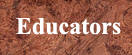Cybrary Man's Educational Web Sites
The internet catalogue for students, teachers, administrators & parents.

Over 20,000 relevant links personally selected by an educator/author with over 30 years of experience.

 Educators         Parents        Students       General     Home
Cybrary Man's Homework SystemI used this homework system during the course of my
32-year teaching career. It is an infallible system if used correctly. No child could ever state that they did not know the homework assignment. No papers were ever lost. Students were rewarded for doing the homeworks and penalized for not handing in homeworks. I did not give students a grade for the homework assignment.

We were required to give daily homework assignments.

My daily homework assignment was to either read, watch or listen to the important news items
and be ready to discuss them the next day. See:
Letter from former student

 Homework SystemHomework Grading System For each marking quarter the students received a test grade for homeworks. If the student completed all the homework assignments on time they received a grade of 100. For some students that was the only 100 they got all term. The homework grade was averaged in with their test scores. Homework assignments handed in late were given half scores. In my gradebook I would put a checkmark for each homework handed in on time. Late homeworks were denoted by the letter “L” in the marking book. Students who were absent were given one day after they returned to hand in the missing homework to get full credit. All homeworks were numbered for that marking quarter. At the end of the marking quarter each homework was given a point value to equal 100. For example: If there were 25 homework assignments for the marking quarter each homework handed in on time was equal to four points. Late homeworks were given 2 points. Missing homeworks were given a zero. Notices and any handouts for absent students were placed in the Homework Folder. Any notices or papers distributed to children who were absent were placed in the folder and given to the absent student when he or she returned to class. Homework after a test was to write the question & correct answer to the questions that they answered incorrectly. (100 on test = no homework) If a student did not understand the homework assignment he/she was required to write a one page summary of what we did in class that day. This way they still had a homework assignment and could not use the "I did not understand" excuse to not do anything. Materials: Two-pocket folders. Use a different colored folder for each class that you teach. Page protector and lined ruled composition paper for each folder. Procedure: In dark magic marker put the name or number of each class on the top right corner of the folder On the left pocket write “Returned Homeworks.” On the right pocket write “Collected Homeworks.” On the composition sheet place the name or number of the class. Homework Monitors For each marking quarter I used three different homework monitors. The “Collected Homework Monitor” would collect all homeworks at the beginning of the period. Students in each class knew that the first thing they had to do when coming into the classroom was to place the completed homework assignment on their desk. The “Returned Homework Monitor” would take the corrected homeworks from the left pocket at the beginning of the class and return them to the students. If the student was absent the monitor would place the paper back in the “Returned Homework” pocket. The “Homework Recording Monitor” would take the page protected paper out and write the number of the homework assignment, date and assignment on the paper. The homework sheet in the page protector was then returned to the folder. Students who had been absent or not sure of an assignment would then take that sheet to copy missing homework assignments and then replace it in the folder. 100% HOMEWORK CLUB At the end of each marking quarter students who completed all of the homeworks on time were given a certificate, a letter to their parent and their names were placed on a chart that hung in the classroom. Homework Help page The Homework Debate page MY19